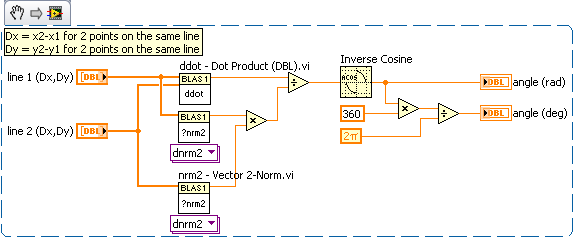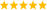# LabVIEW

cancel
Showing results for
Search instead for
Did you mean:

Solved!
Go to solution

## angles between lines

I am trying to figure out a way to figure out the angle between to lines. These lines will always intersect. For example, if one line has the xy values of 100,0 and 100,200 and the other line has an xy value of 0,50 and 200,50. These two lines when ploted on a graph are 90 degrees from each other. I just cant figure out a way to do this. Any ideas?
Message 1 of 16
(4,044 Views)

## Re: angles between lines

From the Analytic Geometry sections of some math handbooks:

The equation of a line passing through two points is: (y-y1)/(x-x1) = (y2-y1)/(x2-x1)

The general form of the equation for a straight line is: A*x + B*y + C = 0

The angle omega between two lines A1*x + B1*y +C1 = 0 and A2*x + B2*y + C2 = 0 is tan omega =  (A1*B2 - B1*A2)/(A1*A2 + B1*B2)

You can do the math to convert the equation in two-points form to the general form to get the angle.

Lynn

Message 2 of 16
(4,030 Views)

## Re: angles between lines

That's basic linear algebra. Use the dot product of 2 vectors to find the cosinus of the angle between these vectors.

Ben

--------------------------------------------------
The best way to say thanks is to give kudos!
Message 3 of 16
(4,028 Views)

## Re: angles between lines

I'm still a little confused. do you have any exmples that might help?
Message 4 of 16
(3,994 Views)
Solution
Accepted by topic author Joe_John

## Re: angles between lines

Here is a way to do it. You can also use the math functions to do it as Lynn suggested.

Ben--------------------------------------------------
The best way to say thanks is to give kudos!
Message 5 of 16
(3,987 Views)

## Re: angles between lines

I tried the code as you posted it but for some reason I thought I would be getting 90 degrees on the attached example.
Message 6 of 16
(3,975 Views)

## Re: angles between lines

This is the long way to do it.
Tim
GHSP
Message 7 of 16
(3,966 Views)

## Re: angles between lines

thanks here is my code modified to work
Message 8 of 16
(3,956 Views)

## Re: angles between lines

I am aware that this thread is very old, but things gets much simpler if we use complex math. Try it!(see also)LabVIEW Champion. It all comes together inGCentralWhat does "Engineering Redefined" mean??
Message 9 of 16
(1,411 Views)

## Re: angles between lines

@altenbach wrote:

I am aware that this thread is very old, but things gets much simpler if we use complex math. Try it!

So are you saying that the real solution is to use imaginary numbers?

Bill(Mid-Level minion.)
My support system ensures that I don't look totally incompetent.
Proud to say that I've progressed beyond knowing just enough to be dangerous. I now know enough to know that I have no clue about anything at all.
Humble author of the CLAD Nugget.
Message 10 of 16
(1,363 Views)